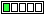All about flooble | fun stuff | Get a free chatterbox | Free JavaScript | Avatarsperplexus dot infoA Quicky II (Posted on 2014-04-03)For a given n what is the smallest number greater than n, not a multiple of n, but containing n in its binary representation?

 No Solution Yet Submitted by Ady TZIDON No RatingComments: ( Back to comment list | You must be logged in to post comments.)re(2): Clarification?| Comment 3 of 7 |Well I suppose it would be the binary representation of (2n+1), but only for those cases where the binary representation of n is divisible by n, otherwise the answer is just the binary representation of n.

Let bin(n) be the decimal number formed by the binary representation of n.

For example, for n = 2, bin(n) = 10.  The smallest number that contains the digits "10" and isn't divisible by 2 is 101, which is the binary representation of 5, which is 2(2) + 1.

On the other hand, for n = 3, bin(n) = 11.  11 is greater than 3, isn't divisible by 3, and contains the digits "11", so that's the solution.

The values n for which bin(n) = 0 mod n are 2, 4, 8, 10, 16, 20, 21, 32, 40, 42, 64, 80, 84, 100, 128, 160, 168, 200, 256, 273...

I don't immediately see any pattern there that would lead to a closed-form solution to your problem for all n.

 Posted by tomarken on 2014-04-03 10:33:09Please log in:

 Search: Search body:
Forums (0)